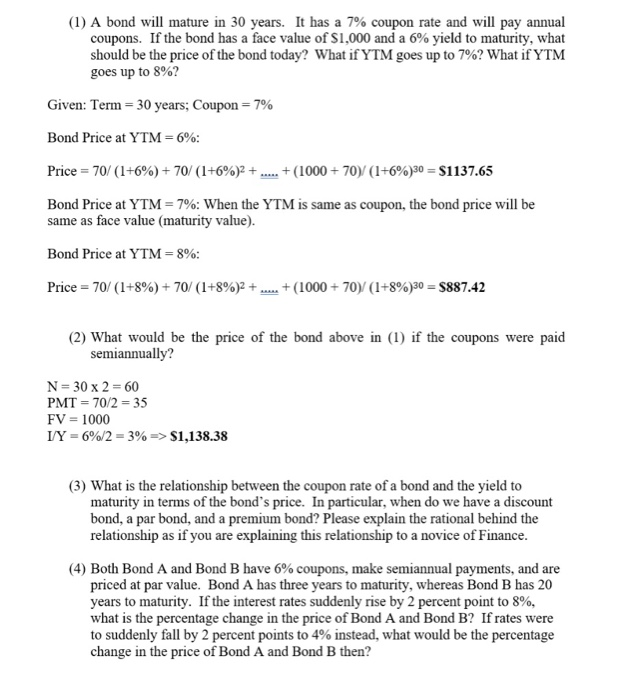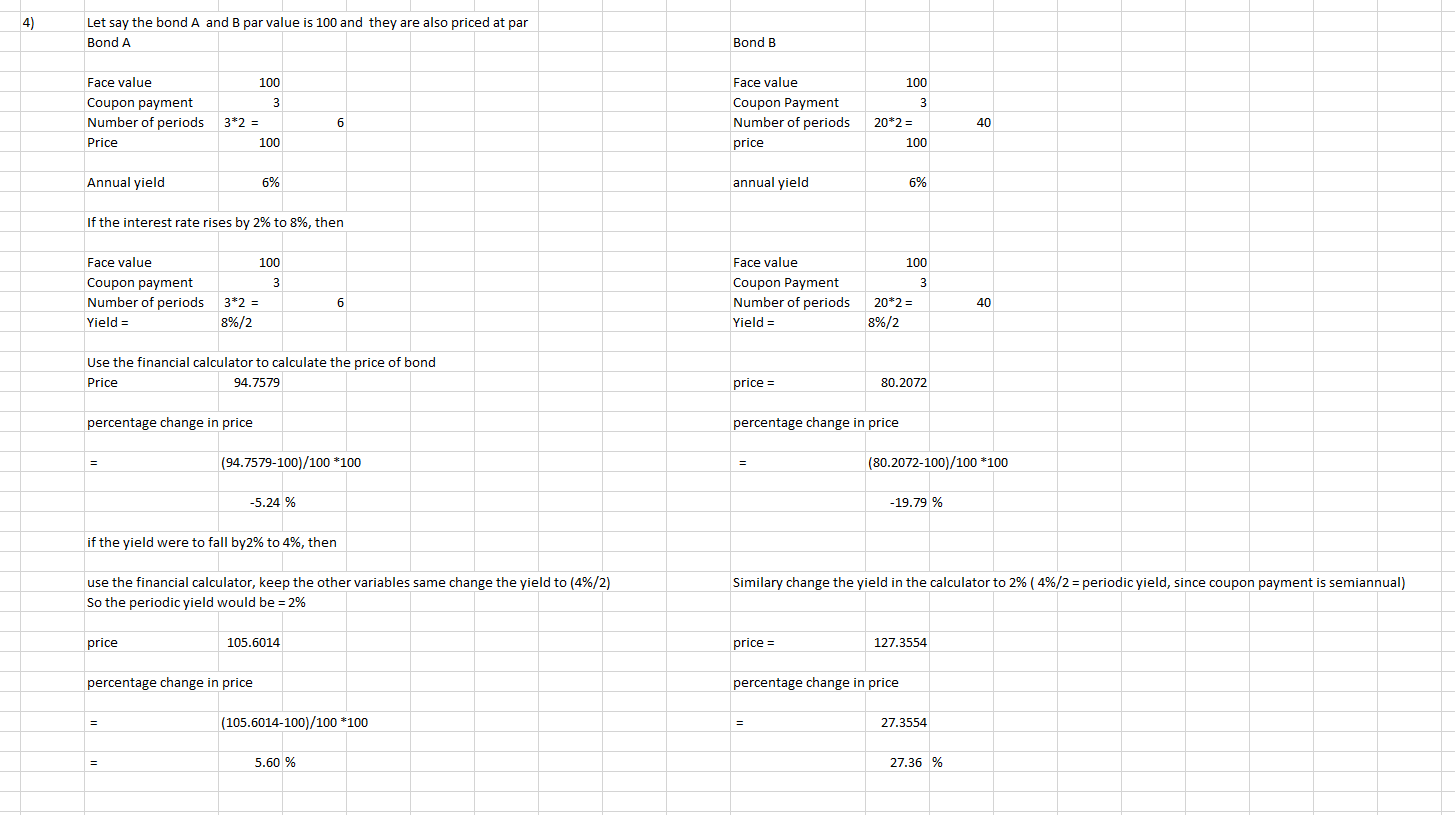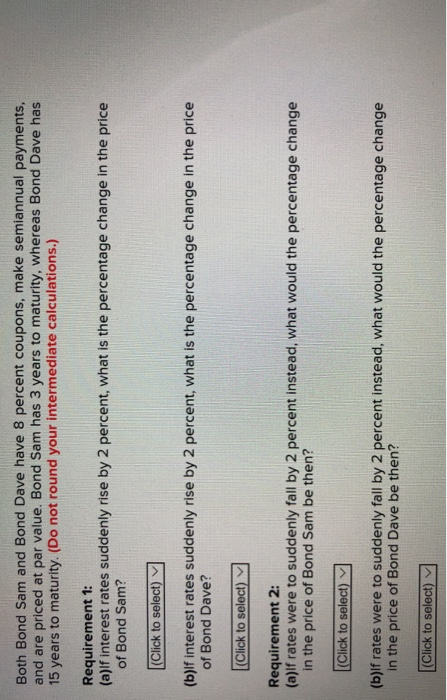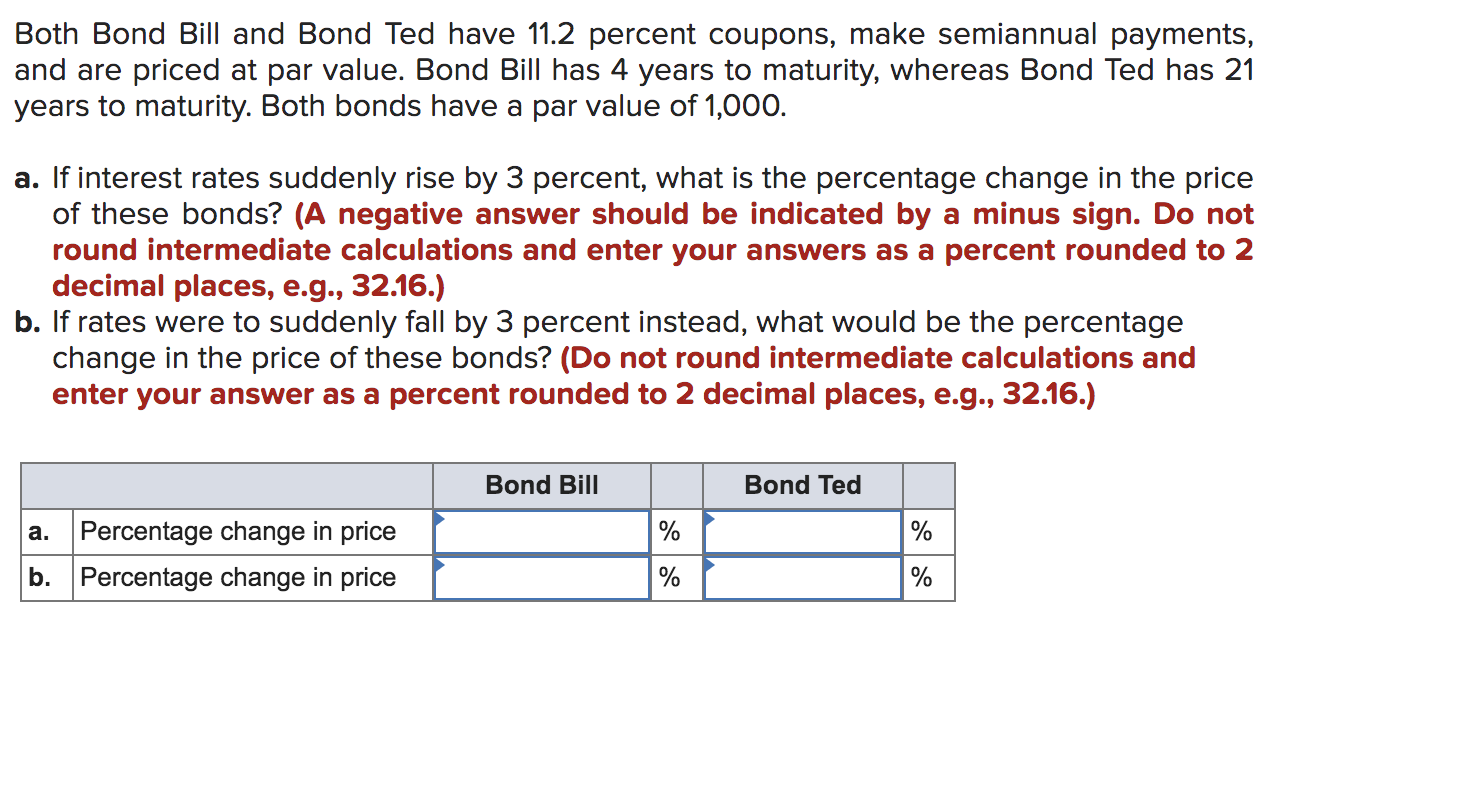Question4. Both Bond A and Bond B have 6% coupons, make semiannual payments, and are priced at par value. Bond A has three years to maturity, whereas Bond B has 20 years to maturity. If the interest rates suddenly rise by 2 percent point to 8%, what is the percentage change in the price of Bond A and Bond B? If rates were to suddenly fall by 2 percent points to 4% instead, what would be the percentage change in the price of Bond A and Bond B then?

QUESTION #4#### Earn Coins

Coins can be redeemed for fabulous gifts.

Similar Homework Help Questions
• ### Both Bond Sam and Bond Dave have 8 percent coupons, make semiannual payments, and are priced...

Both Bond Sam and Bond Dave have 8 percent coupons, make semiannual payments, and are priced at par value. Bond Sam has 3 years to maturity, whereas Bond Dave has 19 years to maturity. (Do not round your intermediate calculations.) Requirement 1: (a) If interest rates suddenly rise by 4 percent, what is the percentage change in the price of Bond Sam? (b) If interest rates suddenly rise by 4 percent, what is the percentage change in the price of...

• ### Both Bond Sam and Bond Dave have 9 percent coupons, make semiannual payments, and are priced...

Both Bond Sam and Bond Dave have 9 percent coupons, make semiannual payments, and are priced at par value. Bond Sam has 5 years to maturity, whereas Bond Dave has 19 years to maturity. Requirement 1: (a)   If interest rates suddenly rise by 2 percent, what is the percentage change in the price of Bond Sam? (b)   If interest rates suddenly rise by 2 percent, what is the percentage change in the price of Bond Dave? Requirement 2: (a)   If...

• ### Both Bond Sam and Bond Dave have 8 percent coupons, make semiannual payments and are priced...Both Bond Sam and Bond Dave have 8 percent coupons, make semiannual payments and are priced at par value. Bond Sam has 3 years to maturity, whereas Bond Dave has 15 years to maturity. (Do not round your intermediate calculations.) Requirement 1: (a)lf interest rates suddenly rise by 2 percent, what is the percentage change in the price of Bond Sam? (b)lf interest rates suddenly rise by 2 percent, what is the percentage change in the price of Bond Dave?...

• ### Both Bond Sam and Bond Dave have 7 percent coupons, make semiannual payments, and are priced...

Both Bond Sam and Bond Dave have 7 percent coupons, make semiannual payments, and are priced at par value. Bond Sam has 3 years to maturity, whereas Bond Dave has 17 years to maturity. (Do not round your intermediate calculations.) Requirement 1: (a) If interest rates suddenly rise by 5 percent, what is the percentage change in the price of Bond Sam? (b) If interest rates suddenly rise by 5 percent, what is the percentage change in the price of...

• ### Both Bond Sam and Bond Dave have 9 percent coupons, make semiannual payments, and are priced...

Both Bond Sam and Bond Dave have 9 percent coupons, make semiannual payments, and are priced at par value. Bond Sam has 5 years to maturity, whereas Bond Dave has 18 years to maturity. 1) If interest rates suddenly rise by 4 percent, what is the percentage change in the price of Bond Sam? 2) If interest rates suddenly rise by 4 percent, what is the percentage change in the price of Bond Dave? 3) If rates were to suddenly...

• ### Both Bond Sam and Bond Dave have 9 percent coupons, make semiannual payments, and are priced...

Both Bond Sam and Bond Dave have 9 percent coupons, make semiannual payments, and are priced at par value. Bond Sam has 4 years to maturity, whereas Bond Dave has 19 years to maturity. If interest rates suddenly rise by 3 percent, what is the percentage change in the price of Bond Sam? If interest rates suddenly rise by 3 percent, what is the percentage change in the price of Bond Dave? If rates were to suddenly fall by 3...

• ### Both Bond Sam and Bond Dave have 6.5 percent coupons, make semiannual payments, and are priced at par value

Both Bond Sam and Bond Dave have 6.5 percent coupons, make semiannual payments, and are priced at par value. Bond Sam has 3 years to maturity, whereas Bond Dave has 20 years to maturity. If interest rates suddenly rise by 2 percent, what is the percentage change in the price of Bond Sam? Of Bond Dave? If rates were to suddenly fall by 2 percent instead, what would the percentage change in the price of Bond Sam be then? Of...

• ### Both Bond Sam and Bond Dave have 9 percent coupons, make semiannual payments, and are priced at par value.

Interest Rate Risk [LO2] Both Bond Sam and Bond Dave have 9 percent coupons, make semiannual payments, and are priced at par value. Bond Sam has 3 years to maturity, whereas Bond Dave has 20 years to maturity. If interest rates suddenly rise by 2 percent, what is the percentage change in the price of Bond Sam? Of Bond Dave? If rates were to suddenly fall by 2 percent instead, what would the percentage change in the price of Bond...

• ### Both Bond Bill and Bond Ted have 11.2 percent coupons, make semiannual payments, and are priced...Both Bond Bill and Bond Ted have 11.2 percent coupons, make semiannual payments, and are priced at par value. Bond Bill has 4 years to maturity, whereas Bond Ted has 21 years to maturity. Both bonds have a par value of 1,000. a. If interest rates suddenly rise by 3 percent, what is the percentage change in the price of these bonds? (A negative answer should be indicated by a minus sign. Do not round intermediate calculations and enter your...

• ### Interest Rate Risk. Both Bond Bill and Bond Ted have 7 percent coupons, make semiannual payments, and are priced at par value.

Chapter 6 Exercise:16 Interest Rate Risk. Both Bond Bill and Bond Ted have 7 percent coupons, make semiannual payments, and are priced at par value. Bond Bill has 3 years to maturity, whereas Bod Ted has 20 years to maturity. If interest rates suddenly rise by 2 percent, what is the percentage change in the price of Bond Bill? Of Bond Ted? If rates were to suddenly fall by 2 percent instead, what would the percentage change in the price of Bond...